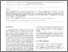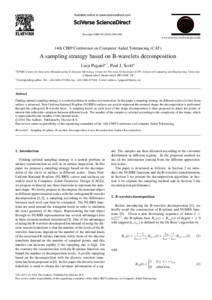Pagani, Luca and Scott, Paul J. (2016) A sampling strategy based on B-wavelets decomposition. Procedia CIRP, 43. pp. 29-34. ISSN 2212-8271
Abstract

Finding optimal sampling strategy is a central problem in surface reconstruction. In this paper a sampling strategy on different scales of a free-form surface is proposed. Non-Uniform Rational B-spline (NURBS) surfaces are used to represent the nominal shape; the decomposition is performed through the orthogonal B-wavelet basis. A sampling based on each level of the shape decomposition is then proposed to detect the points of interest that reflect the variations between different levels. The number of the samples is selected according to the complexity of the shape, which is represented by the number of the internal knots.

Information
Library
DocumentsPreview
A_sampling_strategy_based_on_B-wavelet_decomposition.pdf - Published Version